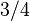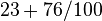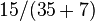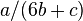# Denominator facts for kids

Kids Encyclopedia Facts

In math, the bottom part of a fraction is called the denominator. A denominator cannot be zero. If the line part of the fraction is flat, the denominator is on the bottom. If the line part is at a slant, the denominator is on the right.The upper part is called the numerator of the fraction. For example:

• In$3/4$ the denominator is "4".
• In$23+76/100$ the denominator is "100".
• In$15/(35+7)$ the denominator is "(35+7)".
• In$a / (6b + c)$, the denominator is "(6b + c)".In Spanish: Fracción para niños